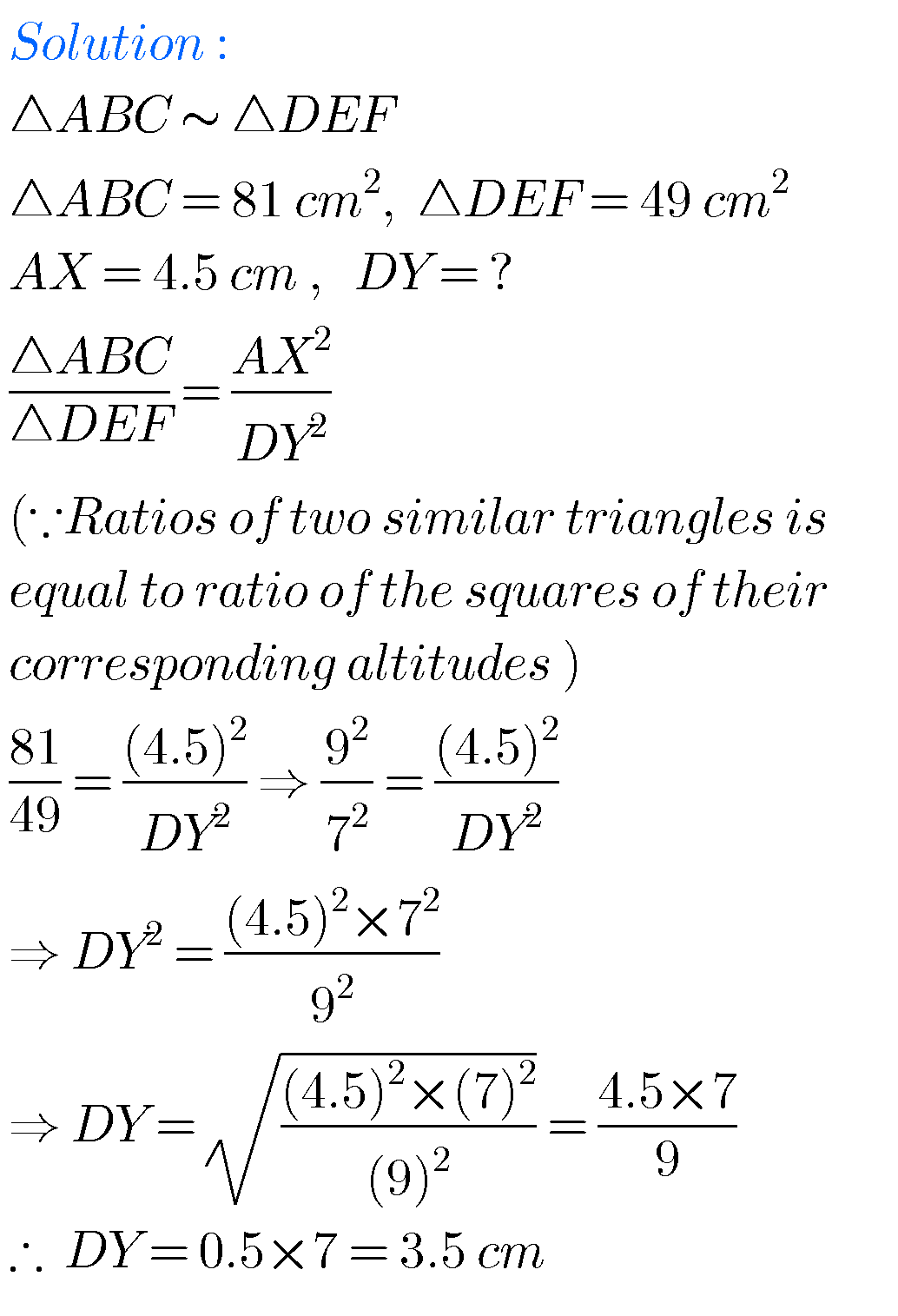## SSC solutions for Maths Similar Triangles class 10

SSC Class 10 solutions for maths Similar Triangles Exercise 8.1,8.2,8.3 and 8.4 Mathematics class 10 SSC Andhra predesh and Telangana Similar Triangles exercise 8.1, exercise 8.2, exercise 8.3 and exercise 8.4 solutions are given. These solutions are very easy to understand. First you study the text book lesson. Observe the example problems and solutions in …